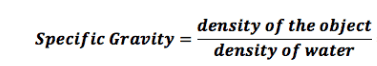# Density and concentrations

wildonion
TL;DR Summary
density, specific gravity, concentrations of salt water
I recently measured the specific gravity of salt water (NaCl) with a hand refractometer of solution to be 1.028 d 20/20 and 36-37 0/00 at 20 deg C. the d 20/20 and 0/00 are the units on the refractometer view screen. I am not sure if the 0/00 means percentage or g/kg.

I am trying to convert this into density (kg/m^3), concentration (mg/L), and (ppm TDS). I am confused on exactly how to do this.

Since the specific gravity is 1.028 that of freshwater, which is 1.000, then density would be 1028 kg/m^3 using the formula below?If the density of 1028 kg/m^3 and I convert units directly, I get something like:

1028 kg/m^3 * 1000000 mg/1kg * 1 m^3 / 1000 L = 1028000 mg/L or 1.028 kg/L

But I have been reading that sea water of approximate density of 1.025 kg/L has approximately 3.5% salt content, 35 g/kg, or 35 000 ppm AND that ppm and mg/L are the same with liquids. So I am really confused why 1028000 mg/L is so much larger. Can anyone point me in the right direction?

I know there is a temperature dependence here too, but I am trying to understand if I am in the ballpark for the concentration value.

Mentor
Do I understand correctly that you compare concentration of the salt to the density of the solution?

wildonion
Borek, thanks for your reply. My goal is to calculate the correct salinity concentration in mg/L from my initial measurement of specific gravity and percentage.

Mentor
The only sure way is to read the concentration for a given density from density tables. Most often they will have density vs w/w %. Once you know density and concentration in any units, you can convert to any other units.

Homework Helper
0/00 means parts per thousand, as 0/0 (%) means parts per hundred. 36 0/00 means a fraction of 0.036, or 3.6%, or 36 g/kg. So your meter is telling you the specific gravity is 1.028 and the salt concentration is 36-37 g/kg, which is consistent with your data for sea water.

Anand Sivaram
What you have written is that you have measured the density of salt water - not sea water, and the difference in density is 1.025 vs 1.028, that is only 0.3% higher.
Could it be due to measurement differences?

You could see that the density of seawater also changes from 1.020 to 1.029
https://en.wikipedia.org/wiki/Seawater#Thermophysical_properties_of_Seawater
Also, the value 3.5% of salt is a kind of average and it varies over different conditions. The Arctic Ocean has a concentration of 3.1% because of ice melting, whereas the Red Sea and the Mediterranean near Egypt has a concentration of 3.8% due to higher evaporation. Freshwater could be seen much further into the open Atlantic Ocean near to the mouth of the Amazon river because of the large volume of freshwater flow.

I just did a bit search, this Britannica article shows 1.02813 as the density at the surface.
https://www.britannica.com/science/seawater/Density-of-seawater-and-pressureAlso, the following ones show different values computed by different calculations
https://hypertextbook.com/facts/2002/EdwardLaValley.shtml

Gold Member
If density is 1.028 g/ml, then surely the mass of salt is 0.028g? Or can someone correct me here.

Anand Sivaram
It depends upon whether the volume changes after salt dissolves.

Mentor
If density is 1.028 g/ml, then surely the mass of salt is 0.028g? Or can someone correct me here.

No, it is not. It is actually quite random and depends on many factors.

Take 2.8 g of NaF, add to 1 L of water - mass is 1028 g, density is 1.0015 g/mL

Take 2.8 g of NaCl, add to 1 L of water - mass is 1028 g, density is 1.0001 g/mL

Take 2.8 g of NaI, add to 1 L of water - mass is 1028 g, density is 1.0003 g/mL

No simple rules that will let you predict the dependence. Experimental tables are the only sure way.

Last edited:
•tech99 and Anand Sivaram
Anand Sivaram
@Borek
Looks like there is a typo... Both (1) and (3) says the same 2.8g of NaF to 1L water but the densities look different.

Mentor
Looks like there is a typo...

Thanks for spotting, yes, should be NaI in the last line. That's what happens when you ^C^V instead of retyping every line.

Corrected.

•Anand Sivaram